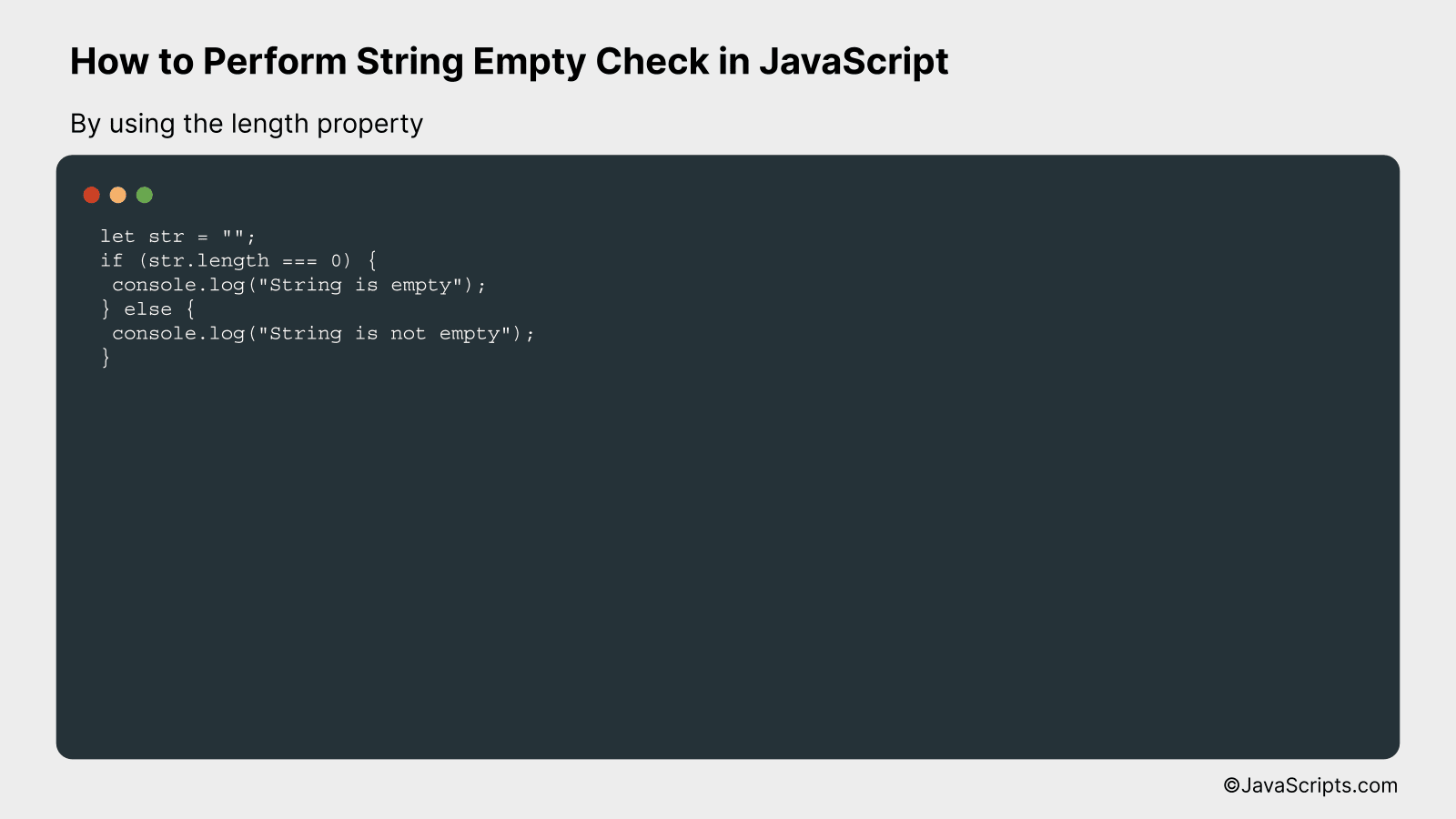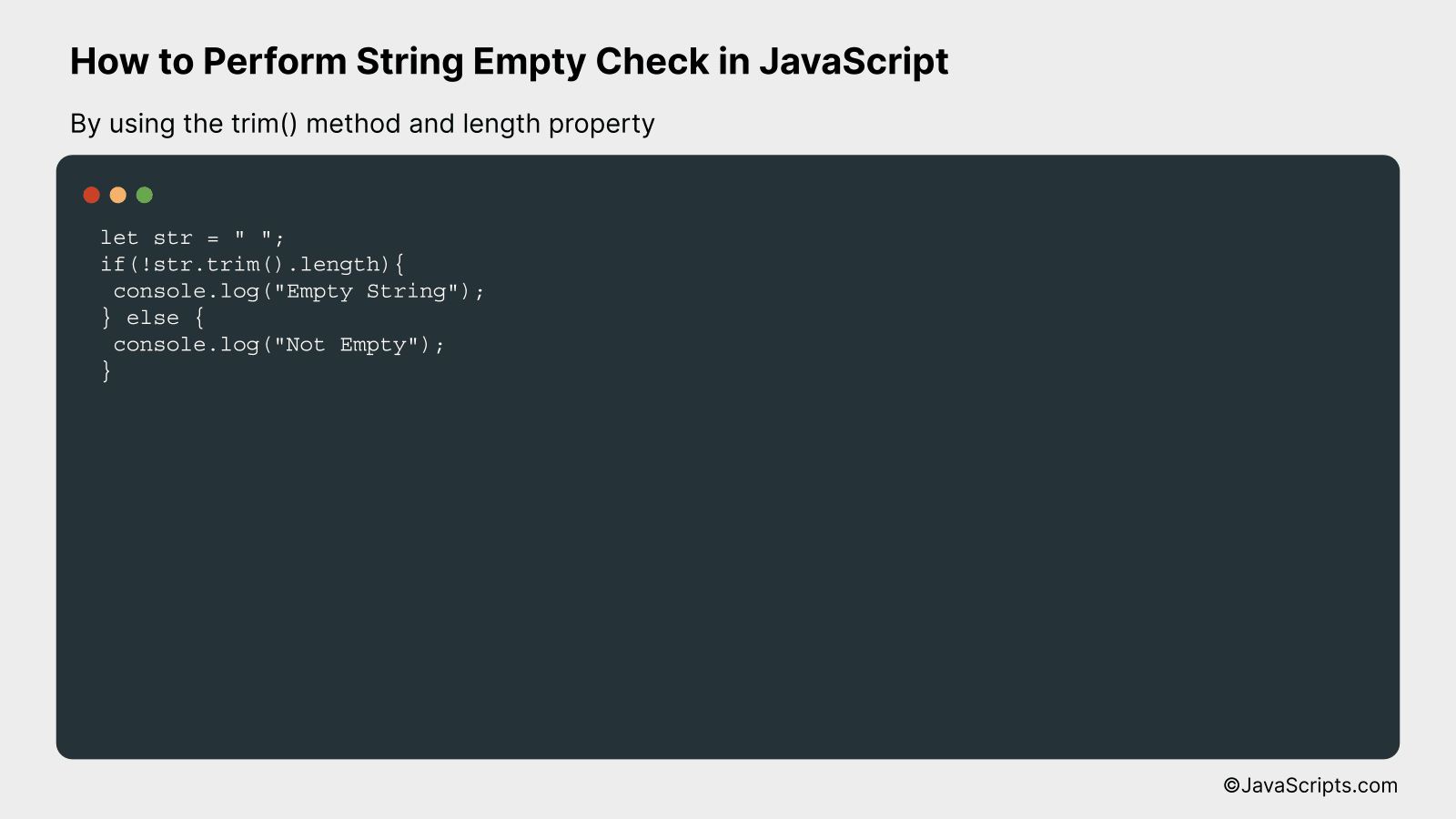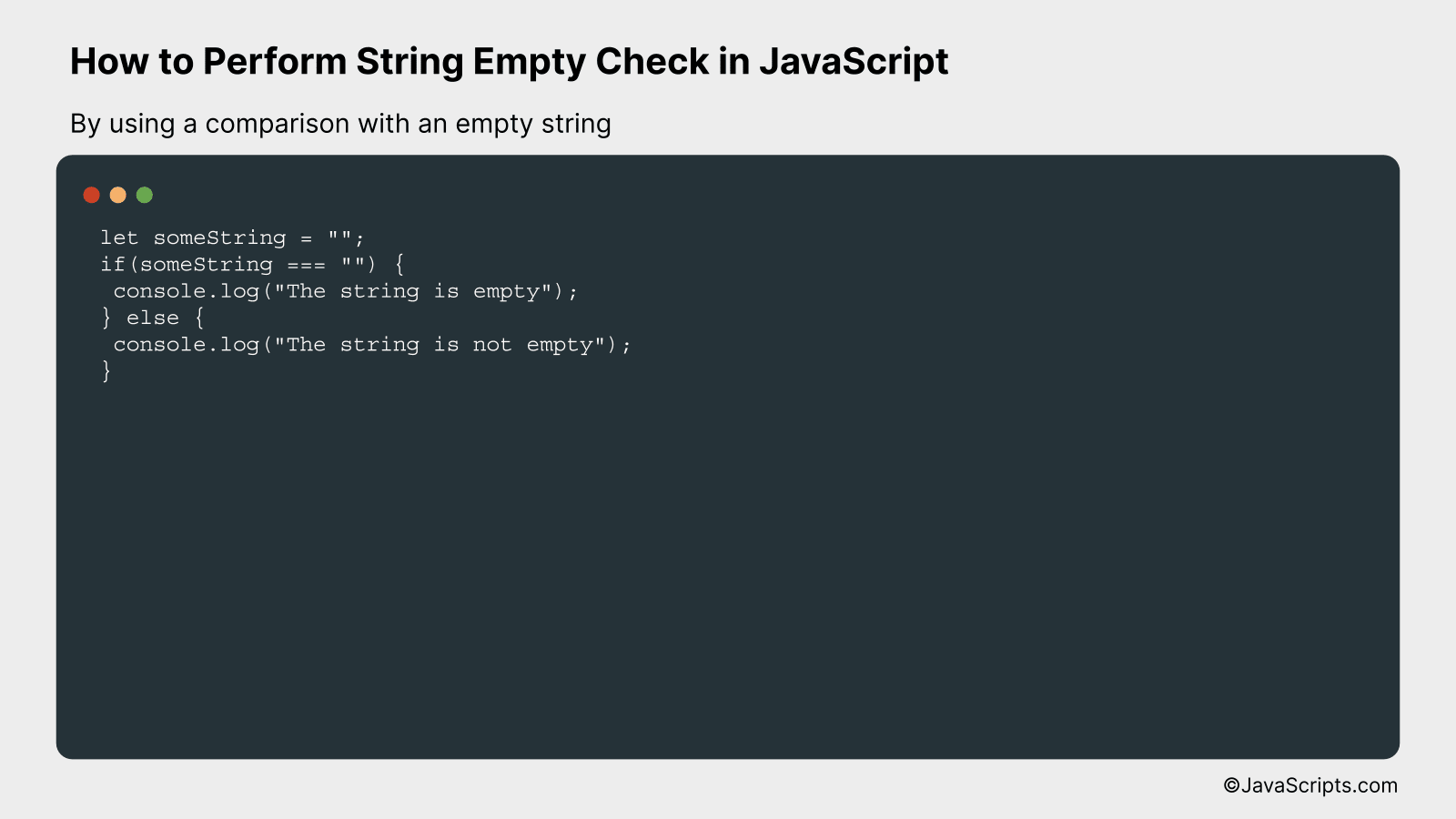# How to Perform String Empty Check in JavaScript

Suppose you’re working on a JavaScript project, and you need to verify whether a string is empty. Sounds simple, right? But, with JavaScript’s dynamic nature, this task isn’t always as straightforward as it seems.

We’ll unravel this conundrum together. After diving into this task, you’ll be able to check for empty strings like a pro, regardless of the complexities that JavaScript might throw your way. Rest easy, we’re about to simplify this important skill for you.

In this post, we will be looking at the following 3 ways to perform string empty check in JavaScript:

• By using the length property
• By using the trim() method and length property
• By using a comparison with an empty string

Let’s explore each…

## #1 – By using the length propertyIn JavaScript, we can check if a string is empty by utilizing the ‘length’ property of the string, which calculates the number of characters present in it. If the length of a string is 0, it signifies that the string is empty. Here is an example of how to implement this.

``````
let str = "";
if (str.length === 0) {
console.log("String is empty");
} else {
console.log("String is not empty");
}
``````

### How it works

The above code checks whether the string is empty or not by comparing the length of the string to zero. If the length is zero, it means the string is empty; otherwise, the string contains some characters.

• The variable ‘str’ is initialized with an empty string.
• Next, the ‘if’ condition checks whether the length of ‘str’ is equal to zero.
• If the condition is true (which means the string length is 0), it logs “String is empty” to the console.
• If the condition is false (which means the string length is not 0), it logs “String is not empty” to the console.

## #2 – By using the trim() method and length propertyThe JavaScript trim() method removes whitespace from both ends of a string and the length property returns the length of a string. By using these two together, we can effectively check if a string is empty (including strings that only contain whitespace). Here’s an example:

``````
let str = " ";
if(!str.trim().length){
console.log("Empty String");
} else {
console.log("Not Empty");
}
``````

### How it works

The trim() method is called on the string, eliminating any leading and trailing whitespace. The length property is then used to check if there are any characters left in the string. If the length is 0, then the string is considered empty.

• First, the trim() function is called on the string variable. This removes any whitespace characters from both ends of the string.
• Next, the length property is used on the trimmed string to find the number of remaining characters. This gives the count of non-whitespace characters in the string.
• If the length of the trimmed string is 0, it means the string was either empty to begin with or only contained whitespace characters. Thus, an if statement is used to check this condition.
• If the length is 0, the log message “Empty String” is printed; otherwise, “Not Empty” is printed.

## #3 – By using a comparison with an empty stringThis method checks if a string is empty by comparing it directly to an empty string (“”). If the string is empty, the comparison results in true, otherwise it results in false. For instance, if we have a string variable, we can directly compare it with an empty string in an if statement, if the condition evaluates to true, then the string is empty.

``````
let someString = "";
if(someString === "") {
console.log("The string is empty");
} else {
console.log("The string is not empty");
}
``````

### How it works

This code works by directly comparing a string variable to an empty string using the strict equality operator (===). If the string variable contains anything other than an empty string, the comparison will evaluate to false. If the string variable is indeed an empty string, the comparison will evaluate to true.

• Firstly, we declare a string variable ‘someString’ and initialize it with an empty string.
• Then, we use an if statement to check if the ‘someString’ variable is strictly equal to an empty string (“”).
• The strict equality operator (===) is used here to ensure not only the value, but also the type is the same. In this case, both the type and value should be an empty string.
• If the comparison is true, the console.log within the if block is executed, which outputs “The string is empty”. This indicates that the string is indeed empty.
• If the comparison is false, the console.log within the else block is executed, printing “The string is not empty”. This indicates that the string contains some value.

Related:

In conclusion, performing an empty string check in JavaScript is a vital skill to have. It helps you validate user input, prevent errors, and keep your applications running smoothly.

Remember, the two main ways to do this are by using the ‘===’ operator or the ‘trim()’ function. Keep practicing, and you’ll soon master these techniques.